@TOC

# # 题目描述1. `rows == heights.length`
2. `columns == heights[i].length`
3. `1 <= rows, columns <= 100`
4. `1 <= heights[i][j] <= 106`

# # 解题思路

## # 并查集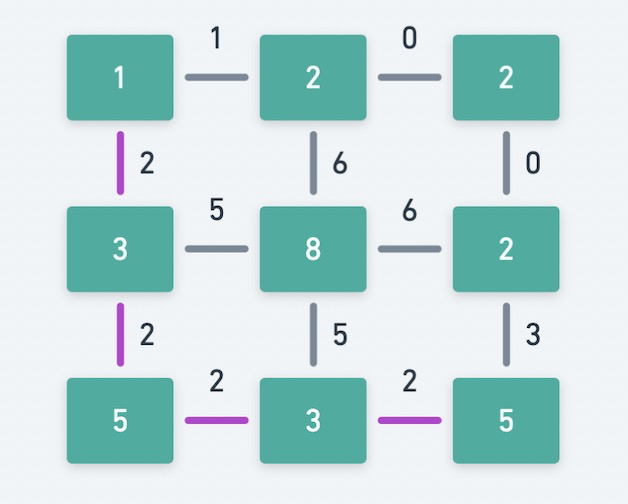1. 最开始，移除所有边。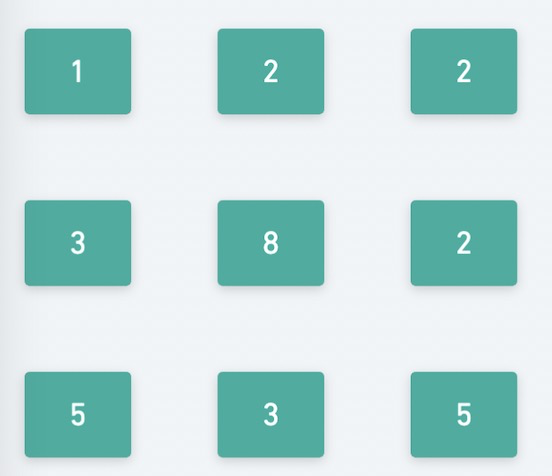2. 然后添加上权重最小的边，即权重为 0 的边。此时的物理含义是判断 0 是不是最小体力消耗值，发现最左上角和最右下角未连通，需要继续。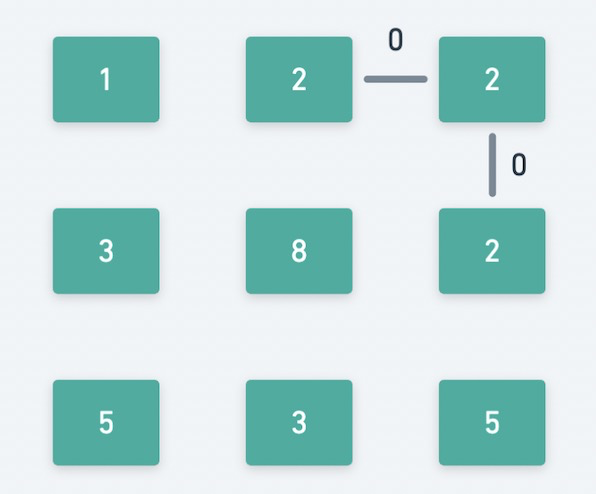3. 然后添加上权重第 2 小的边，即权重为 1 的边。此时的物理含义是判断 1 是不是最小体力消耗值，发现最左上角和最右下角未连通，需要继续。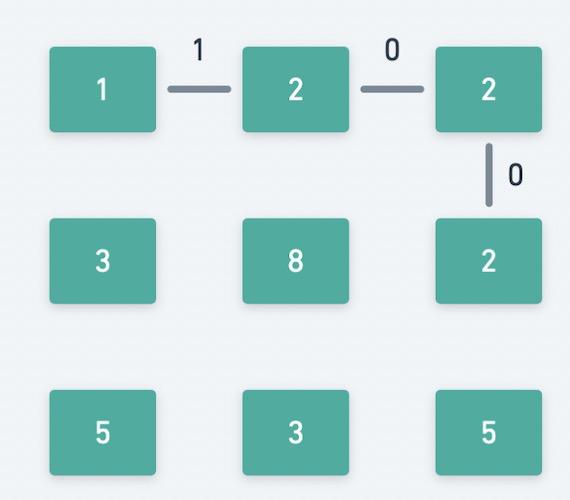4. 然后添加上权重第 3 小的边，即权重为 2 的边。此时的物理含义是判断 2 是不是最小体力消耗值，发现最左上角和最右下角已经连通，找到答案。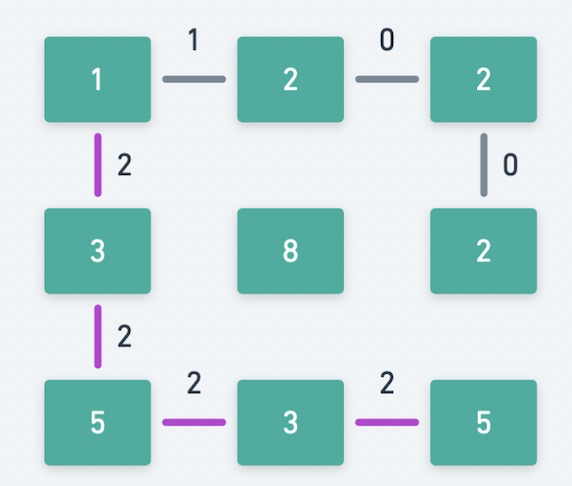# # 代码

1. 首先需要一个并查集的数据结构 DSU，这里直接使用模板。
2. 然后我们需要生成所有的边，并保存到 edges 中。edges[i] 是个 [边的权重，边的第一个顶点，边的第二个顶点] 三元组。把边的权重放在第一位的原因是，我们需要对边的权重排序，在 Python 中调用`sort()`函数，默认会根据第一个元素排。
3. 按照权重对所有的边进行排序`sort()`
4. 遍历所有边，连通这个边的两个节点。并且判断，如果最左上角和最右下角两个节点是否连通了。如果已经连通，则此时的边的权重就是我们要求的最小体力消耗值。

Python2 的代码如下，其他语言可以修改得到。

``````class Solution(object):
def minimumEffortPath(self, heights):
"""
:type heights: List[List[int]]
:rtype: int
"""
M = len(heights)
N = len(heights)
dsu = DSU()
edges = []
for i in range(M):
for j in range(N):
pos = i * N + j
if i < M - 1:
edges.append([abs(heights[i + 1][j] - heights[i][j]), pos, pos + N])
if j < N - 1:
edges.append([abs(heights[i][j + 1] - heights[i][j]), pos, pos + 1])
edges.sort()
for edge in edges:
dsu.union(edge, edge)
if dsu.connected(0, M * N - 1):
return edge
return 0

class DSU:
def __init__(self):
self.par = range(10001)

def find(self, x):
if x != self.par[x]:
self.par[x] = self.find(self.par[x])
return self.par[x]

def union(self, x, y):
self.par[self.find(x)] = self.find(y)

def connected(self, x, y):
return self.find(x) == self.find(y)
``````

# # 刷题心得

OK，这就是本次题解的全部内容了，如果你觉得我的题解对你有帮助的话，求赞、求关注、求转发、求收藏。你的认可就是我前进的最大动力！我们明天再见！

# # 欢迎加入组织# # 日期

2021 年 1 月 28 日 —— 日更公众号的第5天，加油！